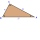# Area of shape + expression of a variable from the formula - math problems

#### Number of problems found: 214

• GardensThe area of the square garden is 3/4 of the area of the triangular garden with sides of 80 m, 50 m, 50 m. How many meters of the fence do we need to fence a square garden?
• Railway embankmentThe section of the railway embankment is an isosceles trapezoid, the sizes of the bases of which are in the ratio 5: 3. The arms have a length of 5 m and the height of the embankment is 4.8 m. Calculates the size of the embankment section area.
• Area and perimeter of rectangleThe content area of the rectangle is 3000 cm2, one dimension is 10 cm larger than the other. Determine the perimeter of the rectangle.
• Extending square gardenMrs. Petrová's garden had the shape of a square with a side length of 15 m. After its enlargement by 64 m2 (square), it had the shape of a square again. How many meters has the length of each side of the garden been extended?
• Sphere in coneA sphere is inscribed in the cone (the intersection of their boundaries consists of a circle and one point). The ratio of the surface of the ball and the contents of the base is 4: 3. A plane passing through the axis of a cone cuts the cone in an isoscele
• HeightThe content of the triangle is 35 cm2. The length of the base is 10 cm. Determine the length of the height on the base.
• Dimensions of the trapezoidOne of the bases of the trapezoid is one-fifth larger than its height, the second base is 1 cm larger than its height. Find the dimensions of the trapezoid if its area is 115 cm2
• Edge of prismThe regular quadrilateral prism has a surface of 250 dm2, its shell has a content of 200 dm2. Calculate its leading edge.
• Cylinder containerThe cylindrical container with a diameter of 1.8 m contains 2,000 liters of water. How high does the water reach?Calculate the content of a regular 15-sides polygon inscribed in a circle with radius r = 4. Express the result to two decimal places.The surface of the regular quadrilateral prism is 8800 cm2, the base edge is 20 cm long. Calculate the volume of the prism
• The circumferenceThe circumference and width of the rectangle are in a ratio of 5: 1. its area is 216cm2. What is its length?
• The tentCalculate how much cover (without a floor) is used to make a tent that has the shape of a regular square pyramid. The edge of the base is 3 m long and the height of the tent is 2 m.Calculate the surface of a quadrilateral pyramid, which has a rectangular base with dimensions a = 8 cm, b = 6 cm and height H = 10 cm.
• Side lengthsIn the triangle ABC, the height to the side a is 6cm. The height to side b is equal to 9 cm. Side "a" is 4 cm longer than side "b". Calculate the side lengths a, b.
• The right triangleThe right triangle ABC has a leg a = 36 cm and an area S = 540 cm2. Calculate the length of the leg b and the median t2 to side b.
• The basesThe bases of the isosceles trapezoid ABCD have lengths of 10 cm and 6 cm. Its arms form an angle α = 50˚ with a longer base. Calculate the circumference and content of the ABCD trapezoid.
• Wallpaper3750 cm square of wallpaper is needed to glue a cube-shaped box. Can Dad cut out the whole necessary piece of wallpaper as a whole if he has a roll of wallpaper 50 cm wide?
• Height to the baseThe triangle area is 35 cm ^ 2. The size of the base is 10 cm. Find the length of height to the base.
• An equilateralAn equilateral triangle is inscribed in a square of side 1 unit long so that it has one common vertex with the square. What is the area of the inscribed triangle?

Do you have an interesting mathematical word problem that you can't solve it? Submit a math problem, and we can try to solve it.

We will send a solution to your e-mail address. Solved examples are also published here. Please enter the e-mail correctly and check whether you don't have a full mailbox.

Please do not submit problems from current active competitions such as Mathematical Olympiad, correspondence seminars etc...